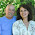Triangle problem with equal areas - solution

This is the solution for the triangle problem with equal areas that I posted earlier.We are given that the areas of the three right triangles are equal, that is the area of the triangle DAE = area of the triangle EBF = area of the triangle FCD.

We will make an equation based on that fact. For that, I like to use x as my variable, so I denote the longer side of the rectangle with a, the other side with b, the distance AE with x, and the distance BF with y. We are asked the ratio AE:EB, which is the same as x : (a − x) using my notation, and the ratio BF:FC, which is the same as y : (b − y) using my notation.

The area of triangle ADE is its base times altitude divided by 2, or bx/2.
The area of triangle EBF is its base times altitude divided by 2, or y(a − x)/2.
The area of triangle CDF is its base times altitude divided by 2, or a(b − y)/2.

And these three are equal. Basically you just make two equations from the above information, and manipulate your equations until you get the ratios expressed without x and y.

Since the area of triangle ADE = area of triangle EBF, we get:

 bx/2 = y(a − x)/2

bx = y(a − x)

bx = ay − yx

Since the area of triangle EBF = area of triangle CDF, we get:

 y(a − x)/2 = a(b − y)/2

y(a − x) = a(b − y)

ay − yx = ab − ay

Now I have two equations with two unknowns (x and y) to solve, or a system of equations:

bx = ay − yx

ay − yx = ab − ay

We'll solve them, and then write the ratios x : (a − x) and y : (b − y).

========================================================

Well, to save time... I did solve these on paper the other day but lost it, and writing it in html is not my favorite hobby... so I just put these equations into Wolframalpha, separated by a semicolon, and got

x = (3a - √5a)/2,   y = (√5 − 1)b / 2

OR

x = (3a + √5a)/2,   y = (√5 − 1)b / 2

Then the two ratios. We need to substitute the value of x from above into the ratio  x : (a − x). I'll use the first solution first.

x : (a − x) = x / (a − x)

= ((3a - √5 a)/2 ) / a − (3a - √5 a)/2)

Hey, maybe Wolframalpha will simplify this expression for me, too. And yes, it did. The simplified form is 1/2 (√5 - 1).

What happens if I use the second solution for x?

In the ratio x / (a − x), we get
(3a + √5a)/2 /(a − ((3a + √5a)/2))

The a's will cancel out from this expression, so we get
(3a + √5a)/2 /(a − ((3a + √5a)/2))

Simplifying with Wolframalpha again, I get (−1 − √5)/2 which is a negative number. So I discard that solution.

Then on to the second ratio, y : (b − y), using the (only) solution y = (√5 − 1)b / 2 first. I substitute that and get ((√5 − 1)b / 2) / (b − ((√5 − 1)b / 2)).

Again, b's cancel out so we have ((√5 − 1)/2) / (1 − ((√5 − 1)/ 2))

Simplifying that, we get (1 + √5)/2, which is the GOLDEN RATIO (or Phi).

So, the two ratios are (√5 + 1)/2 and (√5 − 1)/2, or the golden ratio and its conjugate. Notice these don't depend on a or b (the sides of the rectangle) — meaning that these ratios are the same in any rectangle.Pat B said…
Maria, One of the interesting parts of this problem, as Joshua Z. pointed out in my blog, is that it has a solution at all, that is, that there exists two points as specified which would make the three triangles equal in area, and not only is there one, but it turns out to be at such an interesting proportion of the sides is sort of wonderful.Maria Miller said…
Interestingly to me, it was sort of intuitive (that it has a solution)... I can't explain it totally as to why.

In my mind I was visualizing using a dynamic geometry program and sliding those points... that there should be a point where I can make the areas equal.Josie said…
Great question and really good explanation. I've found a lot of kids really have trouble comprehending the answer if they get a question wrong. If they don't understand why it was wrong they can't learn!Fawn Nguyen said…
This problem is a definite keeper for my geometry kids. Wish we had more time to do these instead of preparing for state tests! UGH!!!That was the nice expatiation of the problem in fact most of the student feel problem in such question as they get confuse in finding the area related problem.